# Population ecology Exponential growth occurs when resources are

• Slides: 15Population ecology Exponential growth occurs when resources are not limiting. Logistic growth occurs when resources become more and more limiting as population size increases.Population ecology • Population growth – Does population growth continue without limits? • Number of resources usually prevent populations from growing exponentially • Carrying capacity (K) = maximum number of individual that an environment can support – When population reaches carrying capacity » birth rate = death rate » population growth rate = 0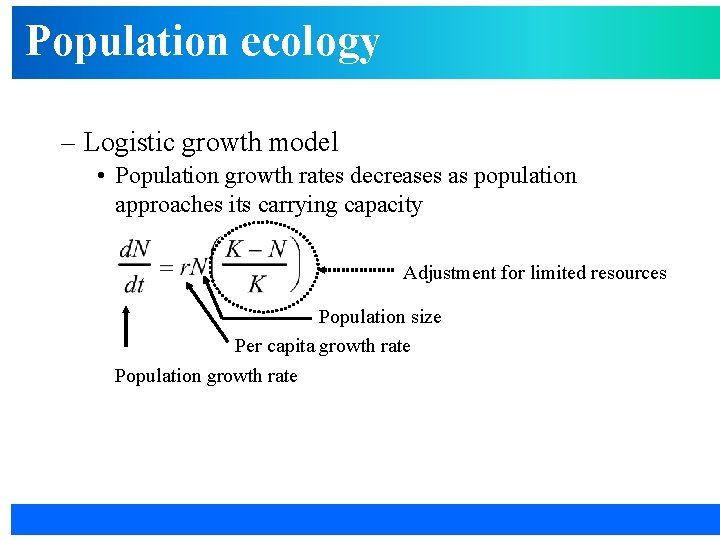Population ecology – Logistic growth model • Population growth rates decreases as population approaches its carrying capacity Adjustment for limited resources Population size Per capita growth rate Population growth ratePopulation ecology • Logistic growth produces S-shaped curve; population growth rate decreases as N approaches K Population size K (N) Time (t)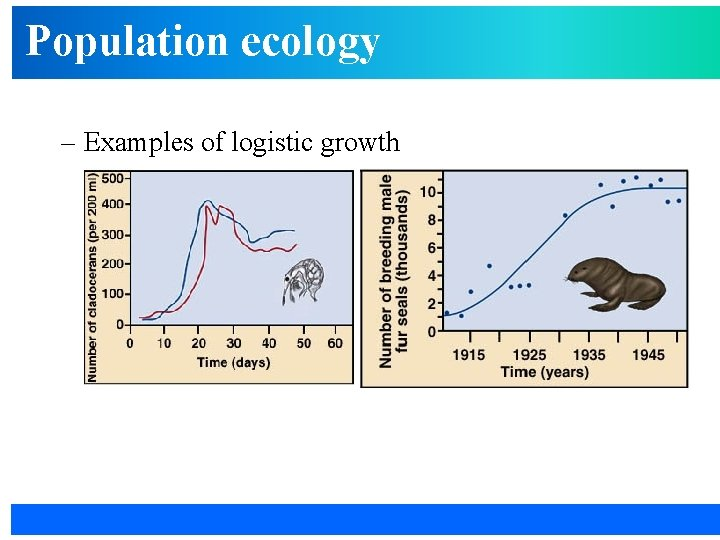Population ecology – Examples of logistic growthPopulation ecology – How does logistic growth model work? • When N is very small (imagine N is 1 and K is 1000). . . is close to 1, so population grows exponentially (1) Population size (N) Time (t)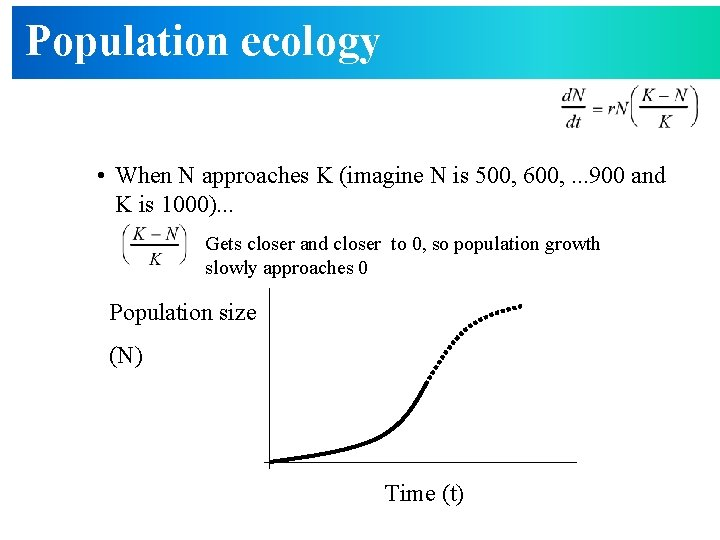Population ecology • When N approaches K (imagine N is 500, 600, . . . 900 and K is 1000). . . Gets closer and closer to 0, so population growth slowly approaches 0 Population size (N) Time (t)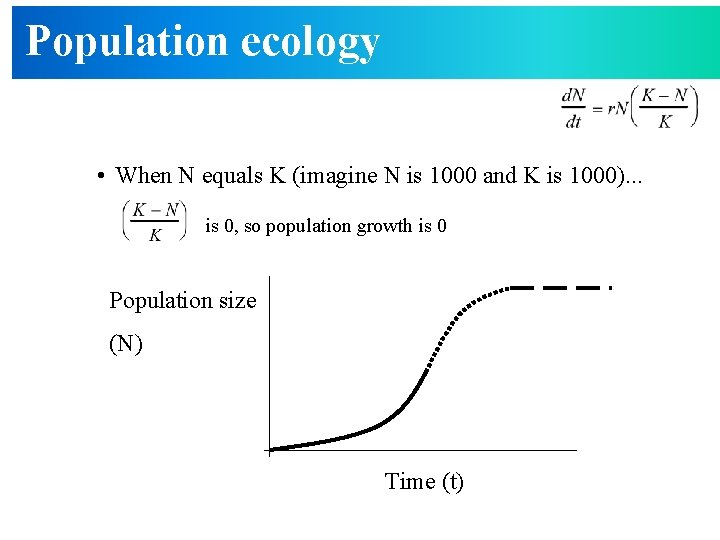Population ecology • When N equals K (imagine N is 1000 and K is 1000). . . is 0, so population growth is 0 Population size (N) Time (t)Population ecology • Population growth – Density-dependent population regulation • As populations near carrying capacity…population growth rate declines – Per capita birth rates decrease (fewer resources available for production of offspring) » fewer resources available for production of offspring – Per capita death rates increase (fewer resources for survival, predators focus attention on common prey) » fewer resources for survival » predators focus attention on common preyPopulation ecology • Population growth – Example of densitydependence • As population size increases, fecundity decreases • As population size increases, mortality increases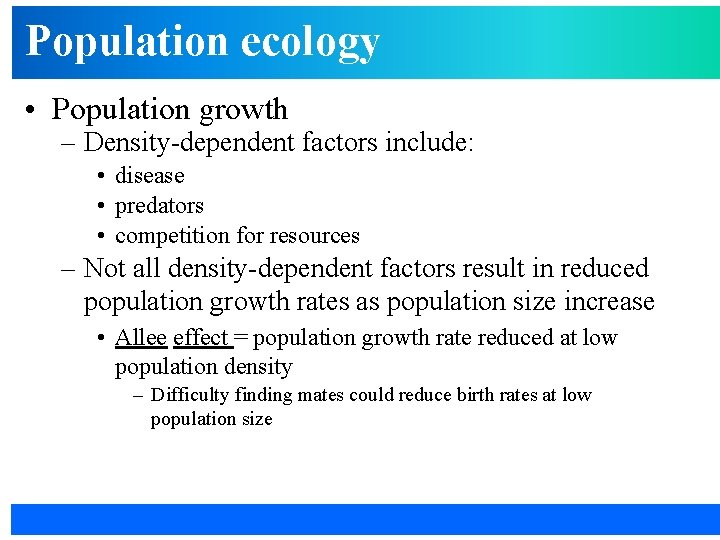Population ecology • Population growth – Density-dependent factors include: • disease • predators • competition for resources – Not all density-dependent factors result in reduced population growth rates as population size increase • Allee effect = population growth rate reduced at low population density – Difficulty finding mates could reduce birth rates at low population sizePopulation ecology • Population growth – Sometimes population regulated by densityindependent factors • Birth rates decrease and death rates increase regardless of population size – Extremely cold winter – drought – fires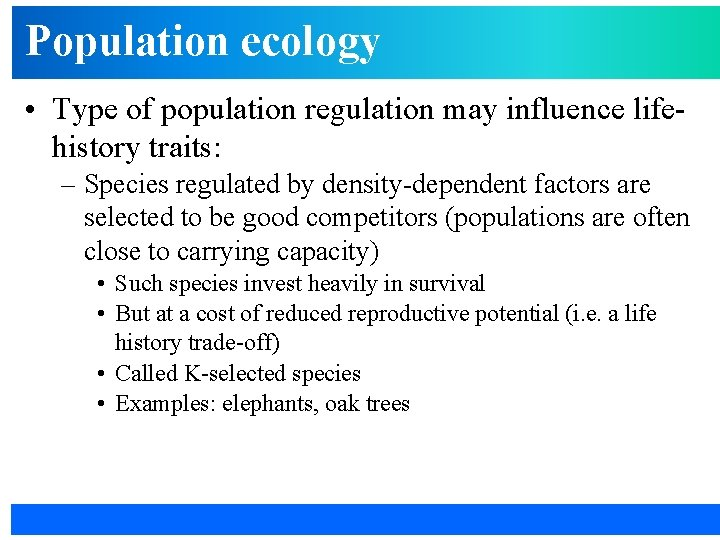Population ecology • Type of population regulation may influence lifehistory traits: – Species regulated by density-dependent factors are selected to be good competitors (populations are often close to carrying capacity) • Such species invest heavily in survival • But at a cost of reduced reproductive potential (i. e. a life history trade-off) • Called K-selected species • Examples: elephants, oak treesPopulation ecology • Type of population regulation may influence lifehistory traits: – Species regulated by density-independent factors are selected to be good reproducers (populations are often below carrying capacity) • • Such species invest heavily in reproductive output But at a cost of reduced survival (i. e. a life history trade-off) Called r-selected species Examples: cockroaches, birch trees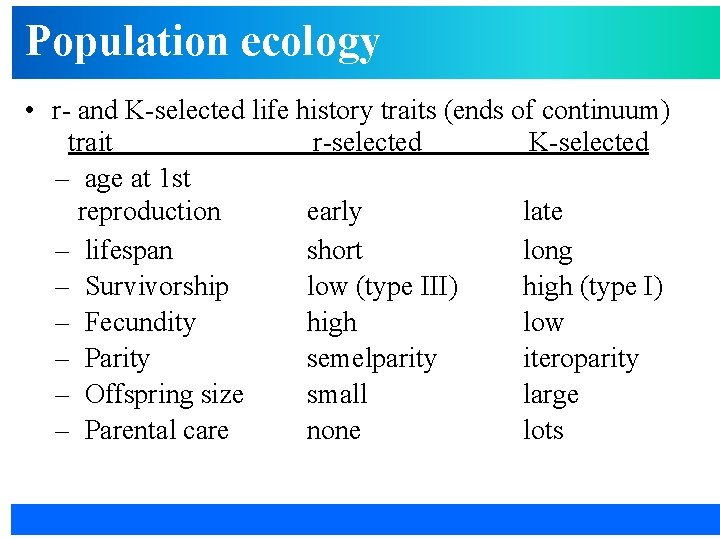Population ecology • r- and K-selected life history traits (ends of continuum) trait r-selected K-selected – age at 1 st reproduction early late – lifespan short long – Survivorship low (type III) high (type I) – Fecundity high low – Parity semelparity iteroparity – Offspring size small large – Parental care none lots